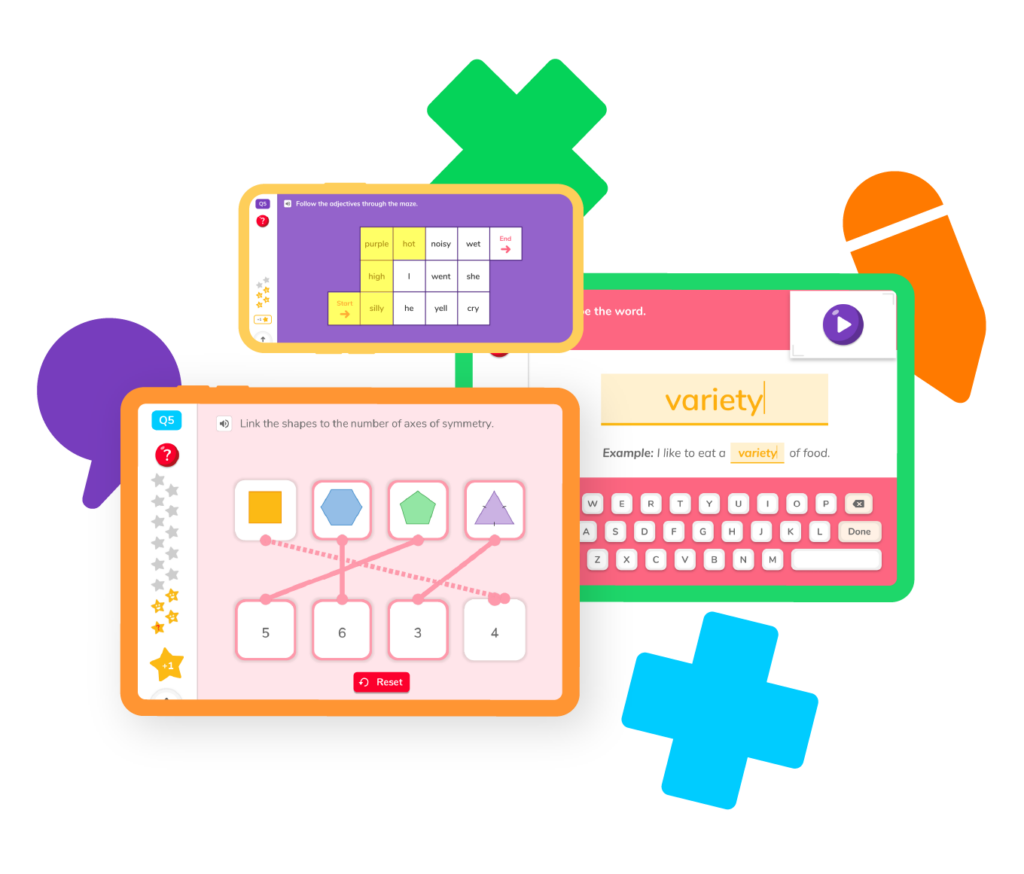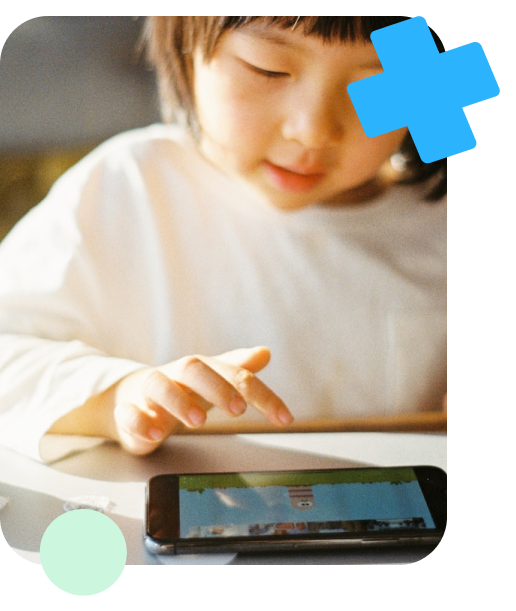# Learning Geometry

Because the world is full of objects, the world is also full of geometryElementary geometry teaches us how to how to identify different shapes, lines, and angles. It teaches us how to use different formulas to calculate the area, perimeter, and volume of shapes and objects, and most importantly how to apply those geometry math concepts to real-world math problems and scenarios.## Learning How to Use Geometry### When should I begin to introduce geometry concepts to my child?

You can begin introducing different types of geometry math concepts to your child as early as kindergarten. You can teach them to identify different shapes based on their features. For example, you can teach them that a triangle has three sides, but a rectangle has four. These are simple concepts that will get them familiar with Geometry.

As your child advances to other grade levels, they will be taught additional Geometry concepts that encompass a wide range of geometry topics including more advanced measurement calculations and more descriptive ways to identify objects. For now, begin with the basics.

## Geometry ResourcesLearning Elementary Geometry can be easy with the right tools. Join us in the Doodle Learning fun! Begin by choosing a Geometry topic. You will discover a variety of Geometry practice problems and learn some of the best tips and tricks from some of the very best teachers and tutors.

## Parallel lines

This article will teach you how to identify parallel lines on a plane.

## Perpendicular lines

Learn characteristics of perpendicular lines and how to classify lines as being perpendicular.

## Area formula

Learn how to find the area of different shapes in Geometry.

## Perimeter formula

Practice finding perimeters of shapes in Geometry and apply this knowledge to real-world examples.

## Volume formula

This article guides you through the steps to find the volume of a cube, rectangular prism, and other common shapes.

## Measuring angles

Learn to use a protractor to measure angles and how to identify acute and obtuse angles.

## Parallel lines

This article will teach you how to identify parallel lines on a plane.

## Perpendicular lines

Learn characteristics of perpendicular lines and how to classify lines as being perpendicular.

## Area formula

Learn how to find the area of different shapes in Geometry.

## Perimeter formula

Practice finding perimeters of shapes in Geometry and apply this knowledge to real-world examples.

## Volume formula

This article guides you through the steps to find the volume of a cube, rectangular prism, and other common shapes.

## Measuring angles

Learn to use a protractor to measure angles and how to identify acute and obtuse angles.

## Veritcies

Discover how to find the vertex of different shapes, angles, and lines.

## Area of a triangle

Discover the steps to calculate the area of any triangle, with practice problems included.When your child is learning Geometry, they will have some questions. Here are the answers to some of the most common questions asked about Geometry.

### What is Geometry?

Elementary geometry is the study of measurements and spatial awareness. It teaches students how to classify shapes, how much space objects take up in the way of volume, area, and perimeter, and how to classify objects based on their lines, angles, and number of sides.

### What are the 3 Types of Geometry?

There are three basic types of Geometry: Euclidean, Elliptical, and Hyperbolic. There are other types of Geometry, but because the other forms are considered a combination of these three, they are not usually included in the list of types of Geometry

### When will you use Geometry in Everyday Life?

Geometry is used almost everyday in our lives. Whenever we fill a glass of milk, or a jug of water, or a pool for summer, we have calculated how much liquid volume that object can hold. That is us using geometry.

We also find the area of objects when trying to calculate how much paint will be needed to cover the area of a wall and when we calculate the perimeter when we want to put a fence around our gardens.

In other words, anytime we calculate a measurement of an object, or classify it based on it’s shape, we are applying the basic concepts of Geometry.

### When do students begin to learn geometry?

Students begin learning geometry in kindergarten, when they are first introduced to shapes and their names. Children are taught to identify shapes based on their characteristics.

As your child advances to fourth grade, they will learn how to draw lines and angles and how to identify shapes by the number of sides.

In fifth-grade math, students will learn how to find measurements including area, perimeter, and volume and will need to apply these skills to real world problems.

### How Can I Supplement My Child’s Learning of Multiplication?

The best way to supplement your child’s learning of Geometry and it’s features is through math practice. There are many resources online that provide geometry math worksheets, games, and quizzes. The more your child is exposed to a variety of geometry practice problems, the more they will be comfortable solving real-world math problems at home and in school.

Check out the best math app for students in grades K-5 to see the many resources we have for students to practice math skills including the Geometry skills in this hub. It also includes a hub for parents to be able to track your child’s progress as they become skilled math learners.

The Geometry Definition is simple: Geometry is the branch in math that applies spatial awareness to real world math problems. These problems include finding the area of objects, calculating the perimeter of different shapes, and identifying lines, vertices, and angles.

You learn Geometry in each grade beginning in kindergarten. Although the concepts develop and become more complex, it is still categorized as geometry when you are learning about shapes, measurements, lines, and angles.

As your child advances to different grade levels, their understanding of Geometry math concepts is broadened. Fifth-grade math has the highest level of geometry math concepts of the grades in elementary. If your child stays on track, these more challenging concepts can easily be learned and practiced.

The three types of Geometry are the Euclidean, Elliptical, and Hyperbolic. Although later forms of Geometry have been added, they are all based on the combination of these three basic types. What is more important than knowing the names of Geometry, is knowing how to apply its concepts. Through much practice, your child can and will succeed.# Are you a parent, teacher or student?

Are you a parent or teacher?

## Hi there!

Book a chat with our team